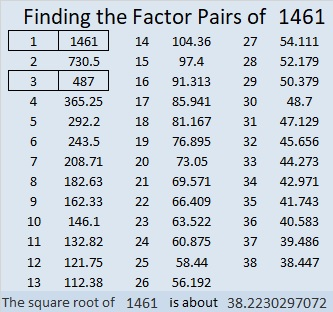# 1461 and Level 6

Contents

### Today’s Puzzle:

Look at all the clues in this level 6 puzzle. Can you deduce the logic needed to begin solving it? Deduce it correctly, then solving the rest of the puzzle will be a breeze.### Factors of 1461:

• 1461 is a composite number.
• Prime factorization: 1461 = 3 × 487
• 1461 has no exponents greater than 1 in its prime factorization, so √1461 cannot be simplified.
• The exponents in the prime factorization are 1, and 1. Adding one to each exponent and multiplying we get (1 + 1)(1 + 1) = 2 × 2 = 4. Therefore 1461 has exactly 4 factors.
• The factors of 1461 are outlined with their factor pair partners in the graphic below.